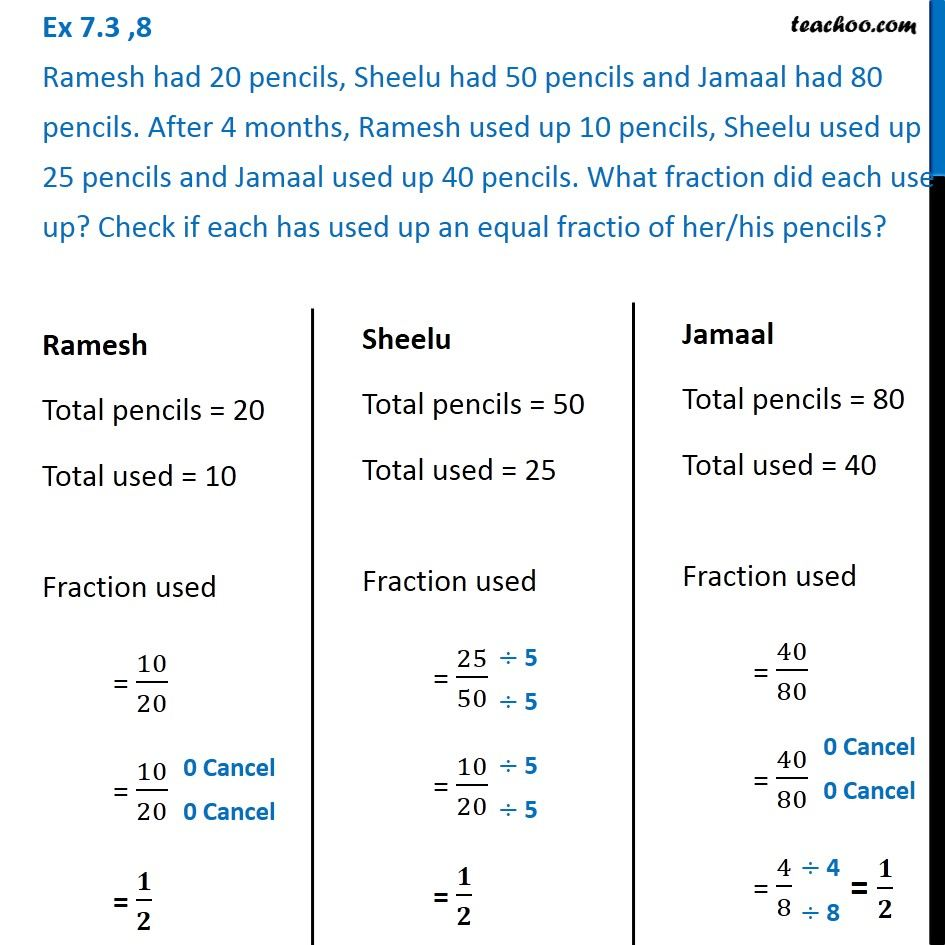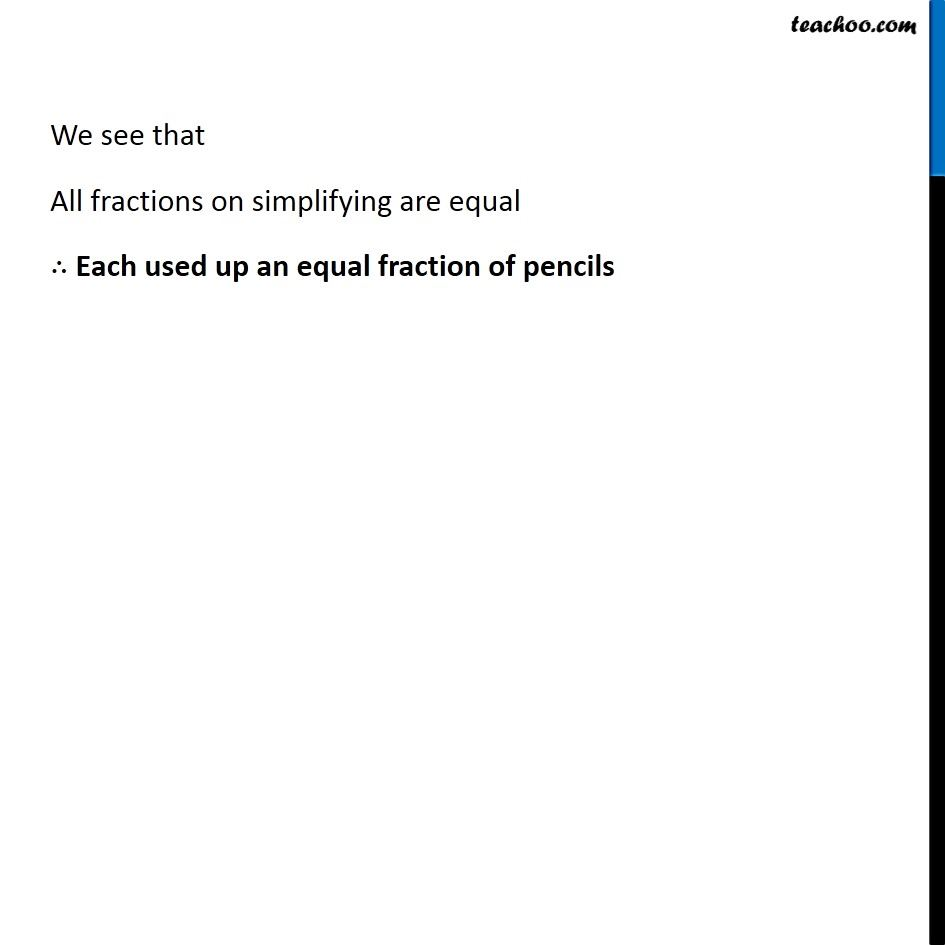Equivalent Fractions

Chapter 7 Class 6 Fractions
Concept wiseIntroducing your new favourite teacher - Teachoo Black, at only ₹83 per month

### Transcript

Ex 7.3 ,8 Ramesh had 20 pencils, Sheelu had 50 pencils and Jamaal had 80 pencils. After 4 months, Ramesh used up 10 pencils, Sheelu used up 25 pencils and Jamaal used up 40 pencils. What fraction did each use up? Check if each has used up an equal fractio of her/his pencils? Ramesh Total pencils = 20 Total used = 10 Fraction used = 10/20 = 10/20 = 𝟏/𝟐 Sheelu Total pencils = 50 Total used = 25 Fraction used = 25/50 = 10/20 = 𝟏/𝟐 Sheelu Total pencils = 50 Total used = 25 Fraction used = 25/50 = 10/20 = 𝟏/𝟐 Jamaal Total pencils = 80 Total used = 40 Fraction used = 40/80 = 40/80 = 4/8 We see that All fractions on simplifying are equal ∴ Each used up an equal fraction of pencils